# Resources tagged with: Calculating with ratio & proportion

Filter by: Content type:
Age range:
Challenge level:

### There are 33 results

Broad Topics > Fractions, Decimals, Percentages, Ratio and Proportion > Calculating with ratio & proportion### Eudiometry

##### Age 16 to 18 Challenge Level:

When a mixture of gases burn, will the volume change?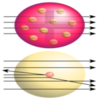### Striking Gold

##### Age 16 to 18 Challenge Level:

Investigate some of the issues raised by Geiger and Marsden's famous scattering experiment in which they fired alpha particles at a sheet of gold.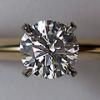### Diamonds Aren't Forever

##### Age 16 to 18 Challenge Level:

Ever wondered what it would be like to vaporise a diamond? Find out inside...### Gassy Information

##### Age 16 to 18 Challenge Level:

Do each of these scenarios allow you fully to deduce the required facts about the reactants?### Why Multiply When You're about to Divide?

##### Age 16 to 18 Challenge Level:

A brief introduction to PCR and restriction mapping, with relevant calculations...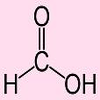### Extreme Dissociation

##### Age 16 to 18 Challenge Level:

In this question we push the pH formula to its theoretical limits.### Investigating the Dilution Series

##### Age 14 to 16 Challenge Level:

Which dilutions can you make using only 10ml pipettes?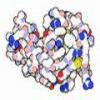### Mixed up Mixture

##### Age 14 to 16 Challenge Level:

Can you fill in the mixed up numbers in this dilution calculation?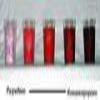### Exact Dilutions

##### Age 14 to 16 Challenge Level:

Which exact dilution ratios can you make using only 2 dilutions?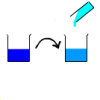### Dilution Series Calculator

##### Age 14 to 16 Challenge Level:

Which dilutions can you make using 10ml pipettes and 100ml measuring cylinders?### Nutrition and Cycling

##### Age 14 to 16 Challenge Level:

Andy wants to cycle from Land's End to John o'Groats. Will he be able to eat enough to keep him going?### Make Your Own Solar System

##### Age 7 to 16 Challenge Level:

Making a scale model of the solar system### Eight Ratios

##### Age 14 to 16 Challenge Level:

Two perpendicular lines lie across each other and the end points are joined to form a quadrilateral. Eight ratios are defined, three are given but five need to be found.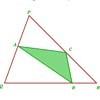### Halving the Triangle

##### Age 16 to 18 Challenge Level:

Draw any triangle PQR. Find points A, B and C, one on each side of the triangle, such that the area of triangle ABC is a given fraction of the area of triangle PQR.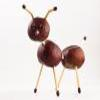### Roasting Old Chestnuts 3

##### Age 11 to 16

Mainly for teachers. More mathematics of yesteryear.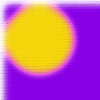### Five Circuits, Seven Spins

##### Age 16 to 18 Challenge Level:

A circular plate rolls inside a rectangular tray making five circuits and rotating about its centre seven times. Find the dimensions of the tray.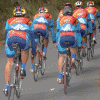### The Fastest Cyclist

##### Age 14 to 16 Challenge Level:

Andy is desperate to reach John o'Groats first. Can you devise a winning race plan?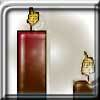### Burning Down

##### Age 14 to 16 Challenge Level:

One night two candles were lit. Can you work out how long each candle was originally?##### Age 14 to 16 Challenge Level:

Two ladders are propped up against facing walls. The end of the first ladder is 10 metres above the foot of the first wall. The end of the second ladder is 5 metres above the foot of the second. . . .### Areas and Ratios

##### Age 16 to 18 Challenge Level:

Do you have enough information to work out the area of the shaded quadrilateral?### Plane to See

##### Age 16 to 18 Challenge Level:

P is the midpoint of an edge of a cube and Q divides another edge in the ratio 1 to 4. Find the ratio of the volumes of the two pieces of the cube cut by a plane through PQ and a vertex.### Golden Ratio

##### Age 16 to 18 Challenge Level:

Solve an equation involving the Golden Ratio phi where the unknown occurs as a power of phi.### Chord

##### Age 16 to 18 Challenge Level:

Equal touching circles have centres on a line. From a point of this line on a circle, a tangent is drawn to the farthest circle. Find the lengths of chords where the line cuts the other circles.##### Age 14 to 16 Challenge Level:

Find the area of the shaded region created by the two overlapping triangles in terms of a and b?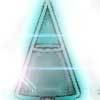### Conical Bottle

##### Age 14 to 16 Challenge Level:

A right circular cone is filled with liquid to a depth of half its vertical height. The cone is inverted. How high up the vertical height of the cone will the liquid rise?##### Age 14 to 16 Challenge Level:

Four jewellers share their stock. Can you work out the relative values of their gems?### Wedge on Wedge

##### Age 14 to 16 Challenge Level:

Two right-angled triangles are connected together as part of a structure. An object is dropped from the top of the green triangle where does it pass the base of the blue triangle?### Arrh!

##### Age 14 to 16 Challenge Level:

Triangle ABC is equilateral. D, the midpoint of BC, is the centre of the semi-circle whose radius is R which touches AB and AC, as well as a smaller circle with radius r which also touches AB and AC. . . .### Contact

##### Age 14 to 16 Challenge Level:

A circular plate rolls in contact with the sides of a rectangular tray. How much of its circumference comes into contact with the sides of the tray when it rolls around one circuit?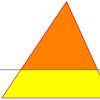### Half a Triangle

##### Age 14 to 16 Challenge Level:

Construct a line parallel to one side of a triangle so that the triangle is divided into two equal areas.### Napoleon's Hat

##### Age 16 to 18 Challenge Level:

Three equilateral triangles ABC, AYX and XZB are drawn with the point X a moveable point on AB. The points P, Q and R are the centres of the three triangles. What can you say about triangle PQR?### Bus Stop

##### Age 14 to 16 Challenge Level:

Two buses leave at the same time from two towns Shipton and Veston on the same long road, travelling towards each other. At each mile along the road are milestones. The buses' speeds are constant. . . .### At a Glance

##### Age 14 to 16 Challenge Level:

The area of a regular pentagon looks about twice as a big as the pentangle star drawn within it. Is it?